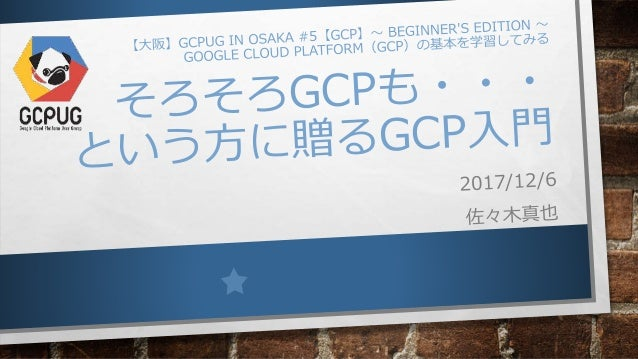Successfully reported this slideshow.Upcoming SlideShare
×

# そろそろGCPも・・・ という方に贈るGCP入門

1,082 views

Published on

【大阪】GCPUG in Osaka #5【GCP】～ Beginner's Edition ～

Published in: Technology
• Full Name
Comment goes here.

Are you sure you want to Yes No• Be the first to like this

### そろそろGCPも・・・ という方に贈るGCP入門

1. 1. モチベーション •マルチクラウドの波 •DR、バックアップ •クラウドインフラのコモディティ化 •強みのあるサービスの利用 •クラウドインテグレータとして •他のクラウドについて聞かれることが増えた •サービスの組み合わせの提案や、最適なサービス選定の必要性
2. 2. GCPってどんなもん？
5. 5. サービスの比較(1)
6. 6. サービスの比較(2)
7. 7. GCPの特徴的なサービス •APP ENGINE (GAE) •KUBERNETES ENGINE (GKE) •BIGQUERY •MACHINE LEARNING
8. 8. COMPUTE ENGINE (GCE)
9. 9. GCEいいところ 1 アカウント ＆ プロジェクト管理
10. 10. ユーザーアカウント •始める時は、普段使って いるGOOGLEアカウン トでログインし、カード 登録するだけ
11. 11. プロジェクト • 作成するリソースはプロジェクト に紐付け • プロジェクト単位でユーザーアカ ウントに権限を付与 • ユーザーアカウント1つですべ てのプロジェクトにログイン できる • プロジェクトを削除すれば全リ ソースも一緒に削除される https://cloud.google.com/iam/docs/resource-hierarchy-access-control
12. 12. GCEいいところ ２ WEBブラウザで全部操作
13. 13. GCPのアクセス方法 •WEBユーザーインターフェイス •CLOUD SDK / CLOUD SHELL •REST API
14. 14. WEBユーザーインターフェイス
15. 15. CLOUD SHELL •GCPの操作で必要なコマンド群がイン ストールされたLINUXコンテナ •WEBインターフェイスから利用可能
16. 16. インスタンスへのSSHアクセス •インスタンス一覧の画面でSSHを選択 •アカウントにプロジェクトの権限を与えるだけで、ユーザーアカウ ントがすべてのGCEインスタンスに自動登録/設定される •パスワードやSSHキーの管理が不要
17. 17. API EXPLORER •ブラウザからAPIコール
18. 18. GCEいいところ 3 ネットワーク
19. 19. SOFTWARE DEFINED NETWORKING ANDROMEDA
20. 20. IPアドレス構成 •複数リージョンで単一のネットワーク
21. 21. GCPのリージョン内レイテンシ • 同一ゾーン • 別ゾーン icmp_seq=1 ttl=64 time=1.26ms icmp_seq=2 ttl=64 time=0.227ms icmp_seq=3 ttl=64 time=0.187ms icmp_seq=4 ttl=64 time=0.179ms icmp_seq=5 ttl=64 time=0.174ms icmp_seq=6 ttl=64 time=0.193ms icmp_seq=7 ttl=64 time=0.202ms icmp_seq=8 ttl=64 time=0.227ms icmp_seq=9 ttl=64 time=0.218ms icmp_seq=10 ttl=64 time=0.231 ms icmp_seq=1 ttl=64 time=1.45 ms icmp_seq=2 ttl=64 time=0.296 ms icmp_seq=3 ttl=64 time=0.258 ms icmp_seq=4 ttl=64 time=0.243 ms icmp_seq=5 ttl=64 time=0.317 ms icmp_seq=6 ttl=64 time=0.254 ms icmp_seq=7 ttl=64 time=0.292 ms icmp_seq=8 ttl=64 time=0.281 ms icmp_seq=9 ttl=64 time=0.308 ms icmp_seq=10 ttl=64 time=0.263 ms インスタンスタイプ：f1-micro
22. 22. AWSのリージョン内レイテンシ • 同一アベイラビリティゾーン • 別アベイラビリティゾーン icmp_seq=1 ttl=255 time=2.77ms icmp_seq=2 ttl=255 time=2.73ms icmp_seq=3 ttl=255 time=2.73ms icmp_seq=4 ttl=255 time=2.66ms icmp_seq=5 ttl=255 time=3.16ms icmp_seq=6 ttl=255 time=2.81ms icmp_seq=7 ttl=255 time=2.73ms icmp_seq=8 ttl=255 time=2.70ms icmp_seq=9 ttl=255 time=2.68ms icmp_seq=10 ttl=255 time=2.71 ms icmp_seq=1 ttl=255 time=0.700ms icmp_seq=2 ttl=255 time=0.420ms icmp_seq=3 ttl=255 time=0.441ms icmp_seq=4 ttl=255 time=0.440ms icmp_seq=5 ttl=255 time=0.469ms icmp_seq=6 ttl=255 time=0.384ms icmp_seq=7 ttl=255 time=0.406ms icmp_seq=8 ttl=255 time=0.404ms icmp_seq=9 ttl=255 time=0.372ms icmp_seq=10 ttl=255 time=0.500 ms インスタンスタイプ：t2.micro
23. 23. CLOUD LOADBALANCER HTTP(S) 負荷分散 •マルチリージョンのバランシングに対応 • 単一のエニーキャスト IPでサービスを提供 • 最も近いリージョンに分散 •毎秒100万件以上のクエリに対応 • プレウォーミング(事前の暖機)も不要
24. 24. GCEいいところ 4 勝手に継続利用割引
25. 25. 継続利用割引 •1 か月の 25% 以上インスタンスを使用 すると、割引が適用 •月全体で実行するインスタンスに対して は最大30%の割引 •継続利用割引は自動適用
26. 26. 考えられるユースケース •急激なアクセス増が頻繁に発生するサイト •ロードバランサが暖機不要 •グローバル展開が必要なWEBサイト •マルチリージョン対応のロードバランサ •負荷が変動 •長期利用割引
27. 27. つらいとこ・・・ •ドキュメント •とっかかりやすいドキュメントがない •AWSのBLACK BELTみたいなやつが欲しい •サービスの多彩さ •AWSと比べると・・・ •IAMがおおざっぱ •リソースレベルでコントロールできないと要件厳しいと対応できない かも